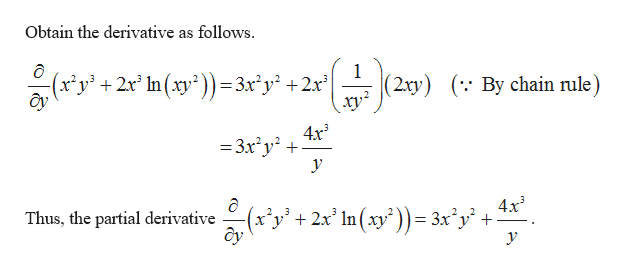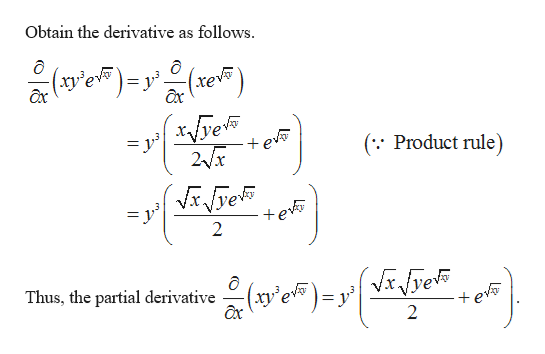Compute the following partialsa) ∂/∂y (x2y3 + 2x3 ln(xy2))b) ∂/∂x (xy3e√(xy))c) ∂/∂y (y2sin(ylnx))

Question

Compute the following partials

a) ∂/∂y (x2y3 + 2x3 ln(xy2))

b) ∂/∂x (xy3e√(xy))

c) ∂/∂y (y2sin(ylnx))

Step 1

(a) The partial derivative is  ∂/∂y (x2y3 + 2x3 ln(xy2).help_outlineImage TranscriptioncloseObtain the derivative as follows 1 (2xy) (y2r In(xy2))=3xy +2r By chain rule 4x3 -3x2y2 4x3 (x'y'+2x' In(xy')) = 3x'y' Thus, the partial derivative fullscreen
Step 2

(b)

Tha partial derivative ∂/∂x (xy3...help_outlineImage TranscriptioncloseObtain the derivative as follows. e ax (se) ax e 2x +ev ( Product rule) te 2 Thus, the partial derivative xy'e ax 2 fullscreen

Want to see the full answer?

See Solution

Want to see this answer and more?

Our solutions are written by experts, many with advanced degrees, and available 24/7

See Solution
Tagged in

Calculus